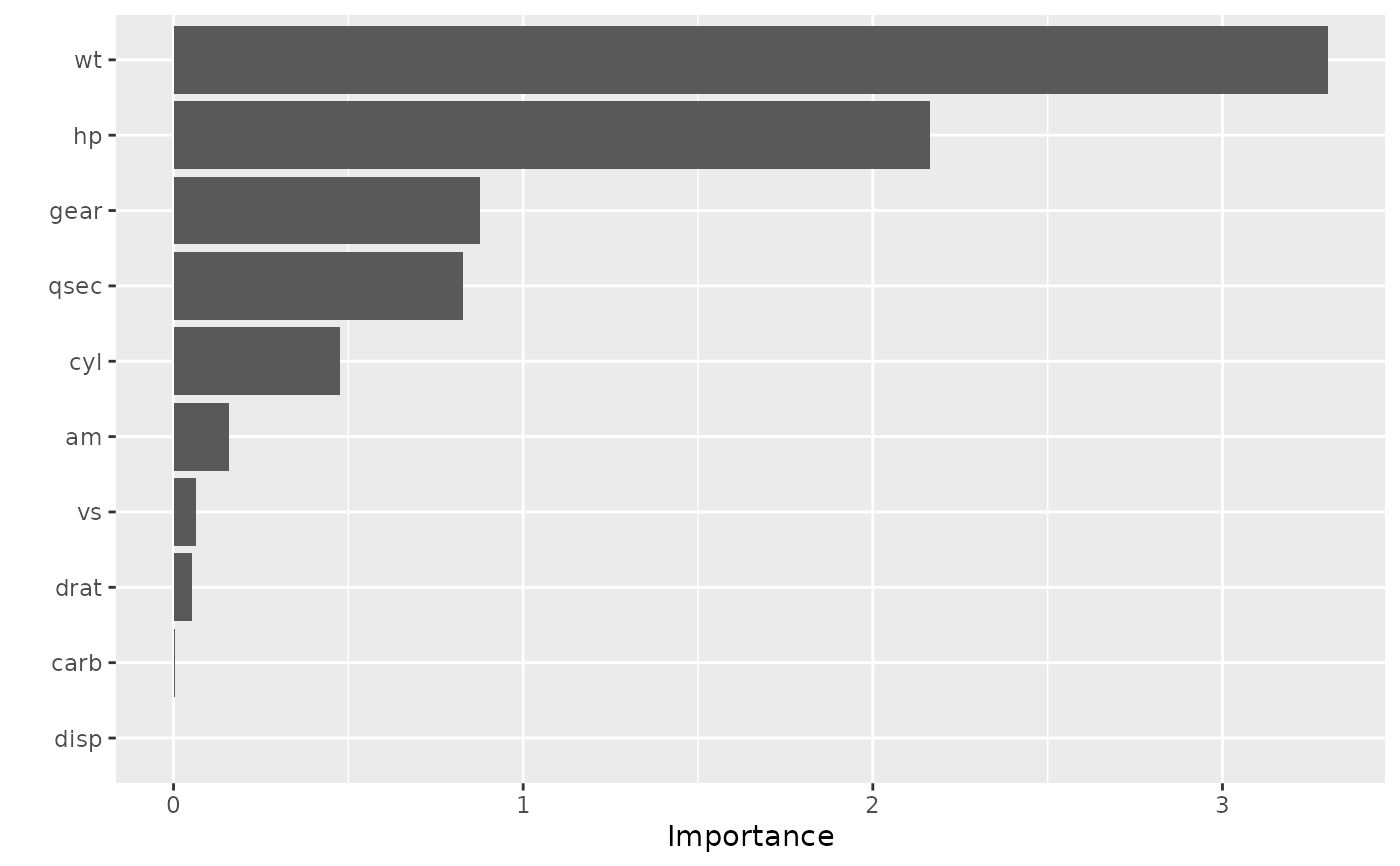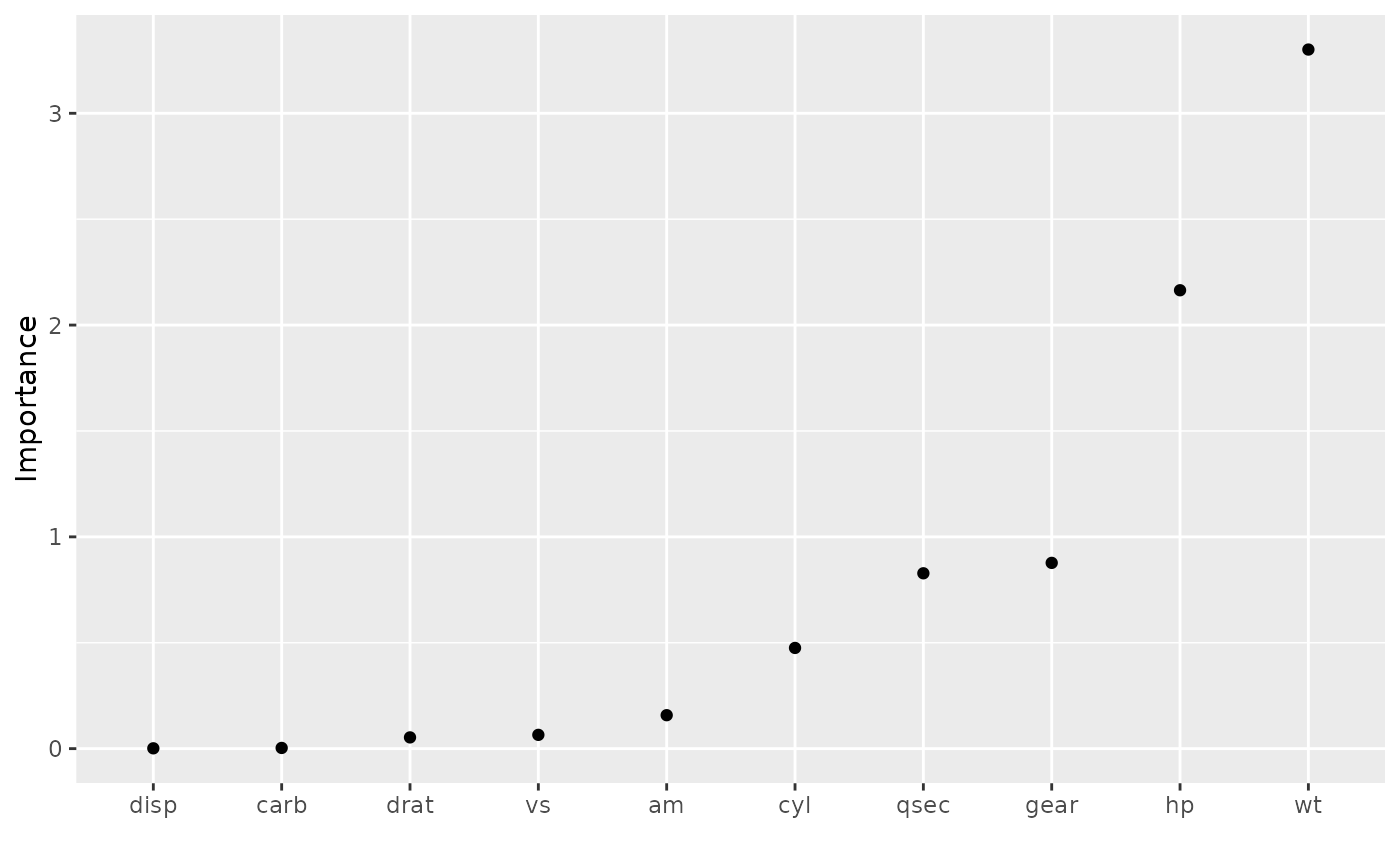Plot variable importance scores for the predictors in a model.

## Usage

vip(object, ...)

# S3 method for default
vip(
object,
num_features = 10L,
geom = c("col", "point", "boxplot", "violin"),
mapping = NULL,
aesthetics = list(),
horizontal = TRUE,
all_permutations = FALSE,
jitter = FALSE,
include_type = FALSE,
...
)

# S3 method for model_fit
vip(object, ...)

# S3 method for workflow
vip(object, ...)

# S3 method for WrappedModel
vip(object, ...)

# S3 method for Learner
vip(object, ...)

## Arguments

object

A fitted model (e.g., of class randomForest object) or a vi object.

...

Additional optional arguments to be passed on to vi.

num_features

Integer specifying the number of variable importance scores to plot. Default is 10.

geom

Character string specifying which type of plot to construct. The currently available options are described below.

• geom = "col" uses geom_col to construct a bar chart of the variable importance scores.

• geom = "point" uses geom_point to construct a Cleveland dot plot of the variable importance scores.

• geom = "boxplot" uses geom_boxplot to construct a boxplot plot of the variable importance scores. This option can only for the permutation-based importance method with nsim > 1 and keep = TRUE; see vi_permute for details.

• geom = "violin" uses geom_violin to construct a violin plot of the variable importance scores. This option can only for the permutation-based importance method with nsim > 1 and keep = TRUE; see vi_permute for details.

mapping

Set of aesthetic mappings created by aes-related functions and/or tidy eval helpers. See example usage below.

aesthetics

List specifying additional arguments passed on to layer. These are often aesthetics, used to set an aesthetic to a fixed value, likecolour = "red" or size = 3. See example usage below.

horizontal

Logical indicating whether or not to plot the importance scores on the x-axis (TRUE). Default is TRUE.

all_permutations

Logical indicating whether or not to plot all permutation scores along with the average. Default is FALSE. (Only used for permutation scores when nsim > 1.)

jitter

Logical indicating whether or not to jitter the raw permutation scores. Default is FALSE. (Only used when all_permutations = TRUE.)

include_type

Logical indicating whether or not to include the type of variable importance computed in the axis label. Default is FALSE.

## Examples

#
# A projection pursuit regression example using permutation-based importance
#

data(mtcars)

# Fit a projection pursuit regression model
model <- ppr(mpg ~ ., data = mtcars, nterms = 1)

# Construct variable importance plot (permutation importance, in this case)
set.seed(825)  # for reproducibility
pfun <- function(object, newdata) predict(object, newdata = newdata)
vip(model, method = "permute", train = mtcars, target = "mpg", nsim = 10,
metric = "rmse", pred_wrapper = pfun)# Better yet, store the variable importance scores and then plot
set.seed(825)  # for reproducibility
vis <- vi(model, method = "permute", train = mtcars, target = "mpg",
nsim = 10, metric = "rmse", pred_wrapper = pfun)
vip(vis, geom = "point", horiz = FALSE)vip(vis, geom = "point", horiz = FALSE, aesthetics = list(size = 3))# Plot unaggregated permutation scores (boxplot colored by feature)
library(ggplot2)  # for aes()-related functions and tidy eval helpers
vip(vis, geom = "boxplot", all_permutations = TRUE, jitter = TRUE,
#mapping = aes_string(fill = "Variable"),   # for ggplot2 (< 3.0.0)
mapping = aes(fill = .data[["Variable"]]),  # for ggplot2 (>= 3.0.0)
aesthetics = list(color = "grey35", size = 0.8))#
# A binary classification example
#
if (FALSE) {
library(rpart)  # for classification and regression trees

# Load Wisconsin breast cancer data; see ?mlbench::BreastCancer for details
data(BreastCancer, package = "mlbench")
bc <- subset(BreastCancer, select = -Id)  # for brevity

# Fit a standard classification tree
set.seed(1032)  # for reproducibility
tree <- rpart(Class ~ ., data = bc, cp = 0)

# Prune using 1-SE rule (e.g., use plotcp(tree) for guidance)
cp <- tree\$cptable
cp <- cp[cp[, "nsplit"] == 2L, "CP"]
tree2 <- prune(tree, cp = cp)  # tree with three splits

# Default tree-based VIP
vip(tree2)

# Computing permutation importance requires a prediction wrapper. For
# classification, the return value depends on the chosen metric; see
# ?vip::vi_permute for details.
pfun <- function(object, newdata) {
# Need vector of predicted class probabilities when using  log-loss metric
predict(object, newdata = newdata, type = "prob")[, "malignant"]
}

# Permutation-based importance (note that only the predictors that show up
# in the final tree have non-zero importance)
set.seed(1046)  # for reproducibility
vip(tree2, method = "permute", nsim = 10, target = "Class",
metric = "logloss", pred_wrapper = pfun, reference_class = "malignant")
}